Parallel transport

Once more, we use the sphere to illustrate how parallel transport works (with the kernel).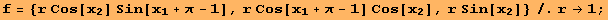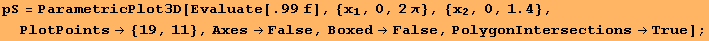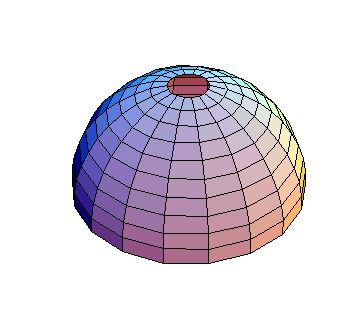The metric in (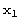,) coordinates is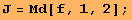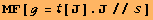The essential computation is carried out in line 1 of the following code. The command is ΧP[g,{t,h},{0,2 π}]. The input to ΧP is the metric g, a curve γ(t):I→M, and the interval I⊂R.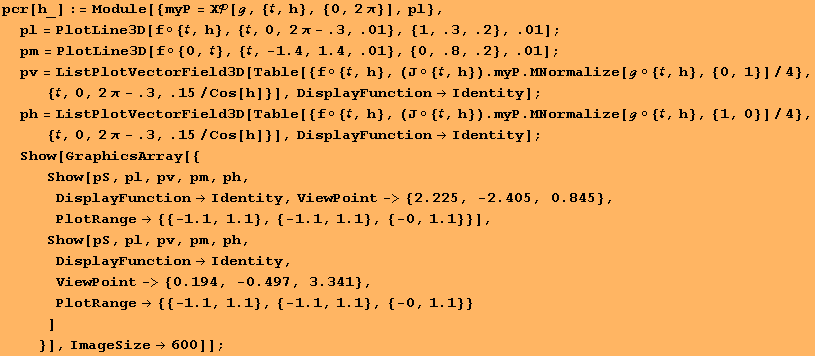We plot parallel transport of an orthonormal frame along several meridians.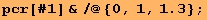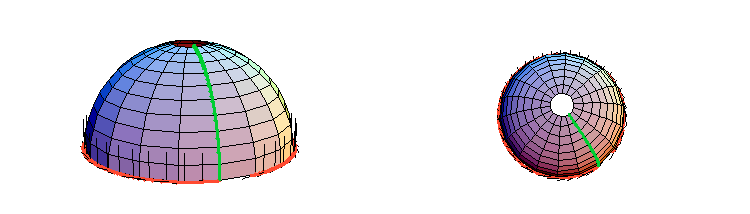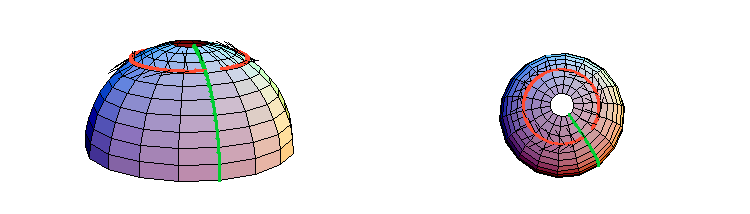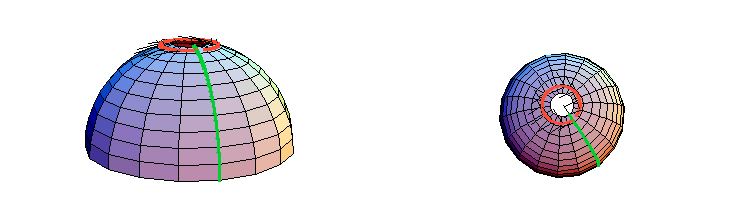An animation is sampled by "AnimationDisplayTime".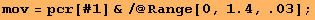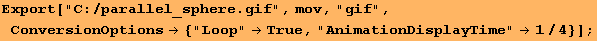Created by Mathematica  (December 22, 2006)